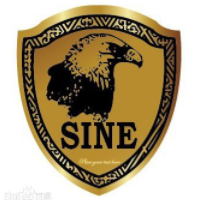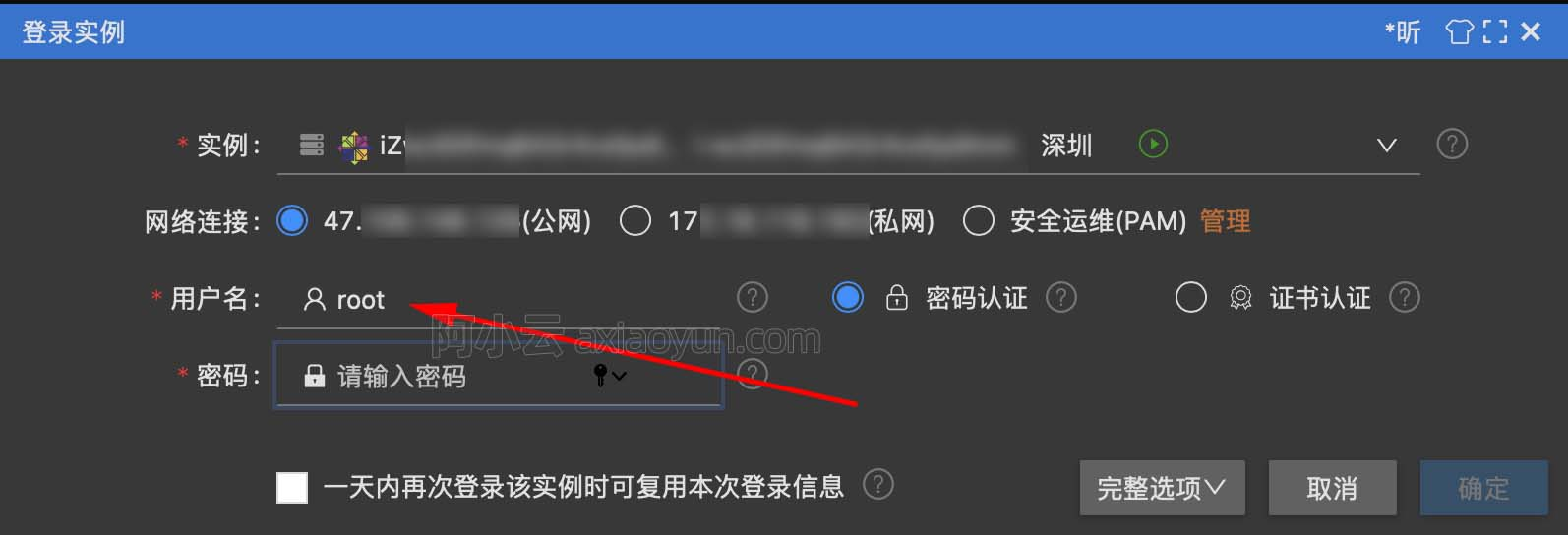【SICP练习】1 练习1.1-练习1.5

+关注继续查看
﻿﻿

(define (sum-of-max x y z)

(cond

((and(<= x y) (<= x z)) (+ y z))

((and(<= y z) (<= y x)) (+ x z))

((and(<= z x) (<= z y)) (+ x y))))

condif在书中都已经有了不少的介绍，在本篇博客之前的文章中对Scheme的基本语法做了一些介绍，对于阅读SICP这本经典作品来说做点准备工作是挺有必要的。

(define (p) (p))

(define (test x y)

(if(= x 0)

0

y))

(test 0 (p))

http://blog.csdn.net/nomasp10062 026784 06762 014483 0windows server 2008阿里云ECS服务器安全设置

9157 013875 04654 07361 04498 054752 0
542

0

《2021云上架构与运维峰会演讲合集》

《零基础CSS入门教程》

《零基础HTML入门教程》